## short communicationsJOURNAL OFSYNCHROTRONRADIATION
ISSN: 1600-5775
Volume 26| Part 1| January 2019| Pages 234-237

## X-ray mirror surface figure correction with nanometre precision controlled by layer stresses simulated by FEA

aLCLS, SLAC National Accelerator Laboratory, 2575 Sand Hill Road, Menlo Park, CA 94025, USA
*Correspondence e-mail: chengxc@slac.stanford.edu

(Received 27 April 2018; accepted 23 October 2018)

Hard X-rays with energies higher than several kiloelectronvolts can be focused to spot sizes below 10 nm with the present synchrotron beamlines, offering unique advantages for the chemical, elemental and structure analysis of matter. Nevertheless, a surface precision on the nanometre scale for the focusing optics is required and remains the main hurdle limiting X-ray analytical techniques with single-nanometre spatial resolution. On the other hand, to preserve the wavefront properties of coherent X-ray beams, precise control of the reflective mirror surface quality at the nanometre scale is demanded for X-ray free-electron laser applications. In this work, the surface shape of a multilayer-coated X-ray mirror is controlled by layer stresses. The desired surface profile of the mirror is differentiated to its second order to obtain its corresponding curvature profile. With a step size of 1 mm along the mirror length, different coating thicknesses are applied to create different layer thermal stresses from uniform temperature change. The mirror surface profile can be obtained by integrating the curvature profile to its second order and further corrected by moving constant values for the slope and height. The technical process is simulated by finite element analysis (FEA). A case study showed that the residual slope error and the residual height error between the desired shape and the FEA result are 0.22 µrad (r.m.s.) and 1.42 nm (r.m.s.), respectively.

### 1. Introduction

Taking advantage of their interactions with matter (diffraction, reflection and refraction), a variety of hard X-ray focusing optical systems such as KB mirrors, zone plates, refractive lenses and multilayer Laue lenses are currently in use (Mimura et al., 2007; Suzuki et al., 2005; Schroer et al., 2005; Kang et al., 2006). Hard X-rays with energies higher than several kiloelectronvolts can be focused to spot sizes below 10 nm, predicted theoretically and realized technically by X-ray reflection optics (Bergemann et al., 2003; Schroer & Lengeler, 2005; Morawe et al., 2008; Mimura et al., 2010). This offers unique advantages for the chemical, elemental and structure analysis of matter with single-nanometre spatial resolution. But a surface precision within the nanometre scale is required for X-ray mirrors which is technically very expensive. On the other hand, for applications making use of X-ray coherence properties such as for X-ray free-electron lasers (XFELs), reflective X-ray mirrors are the main devices for nano-focusing of high-energy X-rays. To preserve the wavefront properties, hard X-ray deformable mirrors must have a surface shape precision at the nanometre level. Cooling techniques such as the matched profile cooling method have been developed to make the most perfect (flat or deformable) mirror surface profile for XFEL high-heat-load optics (Zhang et al., 2015).

At-wavelength metrology methods using short-wavelength X-rays can measure a surface shape with a precision below 1 nm (Mimura et al., 2010). Nevertheless, achieving the atomic level using the present fabrication technologies is very challenging, wherein the mirror can be smoothed and figured flat by elastic emission machining. Aberrations owing to imperfections in the optical system also degrade the quality of the focused beam. Instead of requiring one mirror to be ultimately flat, a twin mirror system with two mirrors consisting of one deformable mirror and one reflective mirror is also applicable. In this scheme, the ideal focusing conditions are achievable even if the X-ray focusing elements do not give sufficient performance. In either way, a precise surface shape control within the nanometre scale is required for X-ray mirrors.

All coated optical elements are in a state of layer stress. Generally, there are two types of layer stress: (i) growth stress (or intrinsic stress) and (ii) thermal stress (Kamminga et al., 2000). Growth stress is caused by the atomic peening mechanism during fabrication processes such as magnetron sputtering. A proper state of growth stress is also necessary to keep the coated layer well attached to the substrate. But the growth stress has very different properties for different types of layer materials and coating conditions. It can hardly be controlled precisely as needed. Thermal stress is due to the thermal misfit between the layer and the substrate materials. It is dilated by cooling of the layer/substrate assembly to room temperature or heating effects from the light source. For multilayer optics, the influences of these coatings on the temperature distribution and thermal deformation are usually negligible as the layers are relatively very thin compared with the substrate. The maximum stress is normally the concerning issue for the damage properties of X-ray multilayer optics such as single-layer coated mirrors or multilayer monochromators. However, for X-ray mirrors nowadays, the height precision of the surface profile is required to be on the nanometre scale, which makes the stress-induced deformation non-negligible. By applying certain layer thickness profiles, the mirror surface shape can also be controlled. In this work, we propose a high-precision surface shape control method for hard X-ray deformable mirrors by utilizing the layer thermal stresses. A particular anomalous surface shape is studied by finite element analysis (FEA) using ANSYS software, giving a quantitative estimation of the effectiveness of this technique. A nanometre precision can be achieved for the residual height error of the mirror shape.

### 2. Method

Multilayer optical elements for hard X-rays are an attractive alternative to crystals whenever high photon flux and moderate energy resolution are required. They typically consist of hundreds of periods of alternating sub-layers coated on a silicon substrate. The thickness of one period of sub-layers is a few nanometres and the multilayer stack can have hundreds of bilayers. As the coefficients of thermal expansion (CTE) of the layer and the substrate materials are mostly different, which is the so-called thermal mismatch, strong stresses can build up in the multilayer when the temperature changes. These layer thermal stresses induce changes in the curvature of the mirror surface.

For the reflection surface of an X-ray mirror, the first-order differential of the mirror surface profile is the slope, which is very much a concern for white-beam optics with high heat-load in a synchrotron beamline. The second-order differential of the mirror surface profile would be its curvature profile. The second-order integral of the curvature curve corresponds linearly to the shape profile but with constant differences for the slope and height. Experimentally these offsets can be further corrected by alignment.

The layer thermal stresses from the thermal mismatch between the substrate and the layer materials induce corresponding curvatures when the temperature changes uniformly. As the layer is much thinner than the substrate, the curvature change can be approximately calculated by equation (1), the Stoney equation (Hsueh, 2002),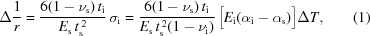where subscripts s and i are for the substrate and the layer, respectively, σi is the layer thermal stress, and E, υ, α and t are the Young's modulus, Poisson's ratio, CTE and thickness, respectively. The thermal stress is proportional to the Young's modulus of the layer material, the difference in CTE between the layer material and the substrate material, and the temperature change. The bending moment created by thermal stress is proportional to the thickness of the layer. The curvature value is the combination of the layer material, the layer thickness and the temperature change. As an example, the substrate of the deformable mirror is assumed to be a silicon block of length 140 mm, width 50 mm and thickness 10 mm, typical sizes for X-ray deformable mirrors. The desired shape profile along the mirror length is shown by the black line in Fig. 1. This profile is similarly re-drawn from Fig. 2of Mimura et al. (2010). Mimura et al. (2010) used a twin mirror KB focusing set-up to focus hard X-rays to a <10 nm spot. The upstream deformable mirror is the key element to correct the wavefront error from the focusing mirror reflection. The desired surface shape of the deformable mirror is anomalous within the nanometre scale, and it is realized by attaching 18 lines of piezoelectric elements on the back of the mirror. Here, we take this profile as a case study; we will realize the profile using layer stresses. The height data are differentiated to the second order to obtain the curvature profile, as shown by the blue curve in Fig. 1.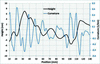Figure 1 Desired shape of the hard X-ray deformable mirror: surface shape (height), shown by the black line, and the curvature profile calculated by differentiating the height data to its second order.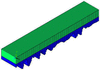Figure 2 Finite element modeling of the mirror; the layer thickness is multiplied by 1000 for a better visualization. The mirror substrate is in green and layer coatings on the back are in purple.

If we applied the coating on the back of the mirror surface, the curvature induced by the layer thermal stress when the temperature rises can only be a positive value when the CTE of the layer material is higher than that of the substrate. So the curvature profile in Fig. 1is moved positively by 0.4 km−1 to make the curvatures all positive. By assuming a uniform temperature rise of 100 K, the applied layer thicknesses can be calculated by the Stoney equation from the corrected curvature profile. The layer material is chosen to be Pd, which is commonly used for reflecting hard X-rays. The material properties of Pd and Si are listed in Table 1.

 Table 1Material properties of Pd and the Si substrate
α (×10−6 K−1) E (GPa) Poisson's ratio ν
Si 2.6 112.4 0.28
Pd 11.1 117 0.39

Many methods can be used to apply a constant curvature value, for example bending and uniform layer coating. In our simulation we form a uniform layer coating on the mirror surface to remove the constant curvature. In this model a curvature value of 0.1 km−1 corresponds to a layer thickness of 1.596 µm. This thickness value is unusual for multilayer optics which generally have a thickness below 1 µm. Technically, a coating thickness of tens of micrometres can be achieved by current magnetron sputtering deposition or other coating techniques. Thick coatings have found specialized applications such as in multilayer Laue lenses (MLLs) but the coating type is limited to certain groups of materials. The step size along the mirror length is 1 mm. The step-profile coating technique is applied with a layer thickness precision of 10 nm, which can be easily achieved by the current coating techniques. The technical process is simulated by FEA by using the multilayer structural model in ANSYS as shown in Fig. 2. The average value for the layer thickness is 6.14 µm and the maximum layer thickness is 13.19 µm. The layer thickness can be reduced by applying a larger temperature change value or choosing a layer material with higher thermal expansion coefficient. For example, if we assume a uniform temperature rise of 500 K, the applied layer thicknesses will be reduced by a factor of five, which makes the average layer thickness 1.228 µm and the maximum layer thickness 2.638 µm. But both cases generate the same layer thermal stresses. The FEA result of the curvature profile is shown in Fig. 3. Spaces of 10 mm are applied to both sides of the mirror length to avoid the end effect. Deposition of a variable thickness up to the micrometre level often requires a gradient in one or both components in the multilayer stack. The coating condition may also strongly alter the intrinsic stress. In the simulation, the intrinsic stress is assumed to be zero but can be increased. This simulation only evaluates the effects from thermal stresses.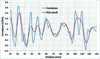Figure 3 Comparison between the original calculation (second-order differential of the shape profile) and the FEA simulation for the curvature.

The mean values of the curvatures are −0.0014 km−1 and 0.0032 km−1, from the original calculation and the FEA simulation, respectively. The root-mean-square (r.m.s.) difference of the curvatures between the original calculation and the FEA simulation is 0.0095 km−1. The slope results from the derivative of the mirror shape profile and the FEA simulation are shown in Fig. 4. The mean values of the slopes for the original calculation and the FEA simulation are 0.05 µrad and −0.19 µrad, respectively. From the integral properties, the FEA result should correspond to the slope calculation but with a constant difference. The FEA slopes are then corrected by adding 0.24 µrad, which is shown by the red curve in Fig. 4. The r.m.s. difference of the slopes between the desired shape and the FEA result is 0.22 µrad within the range 10–130 mm.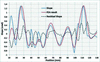Figure 4 Comparison between the desired slope profile and the FEA simulation result. The residual slope is the difference between the FEA data and the desired slopes.

The height data from FEA simulation are shown in Fig. 5. As the FEA slope result is corrected by adding 0.24 µrad and the height data is the integral of the slope, two correction steps are used for the FEA height data. Firstly, the FEA heights are tilted by adding 0.24X, where X is the position coordinate along the mirror length. The mean values of the heights and the corrected FEA result are 0.04 nm and 2588.9 nm, respectively. Secondly, the FEA heights are further corrected by adding 2588.9 nm. The final result is shown by the red curve in Fig. 5. The r.m.s. difference of the heights between the desired surface shape and the corrected FEA result is 1.36 nm within the range 10–130 mm.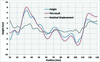Figure 5 Comparison between the desired shape profile and the FEA simulation result. The residual displacement is the difference between the FEA data and the desired shape.

### 3. Conclusion

To achieve a surface precision within the nanometre scale for hard X-ray mirrors, a layer-stress-controlling method is proposed and the technical process is simulated by FEA. A special case-study taking the desired surface shape of the deformable mirror from Mimura et al. (2010) is demonstrated quantitatively giving numerical values for the precision. The mirror shape is firstly differentiated to its second order to obtain the curvature profile. Different layer thicknesses along the mirror length are then determined from the curvature profile and applied to the mirror to create different layer thermal stresses. The step size along the mirror length is 1 mm and the layer thickness precision is taken to be 10 nm. The residual slope error and the residual height error between the desired shape and the FEA result are 0.22 µrad (r.m.s.) and 1.42 nm (r.m.s.), respectively, within the range 10–130 mm of the mirror length.

### ReferencesJOURNAL OFSYNCHROTRONRADIATION